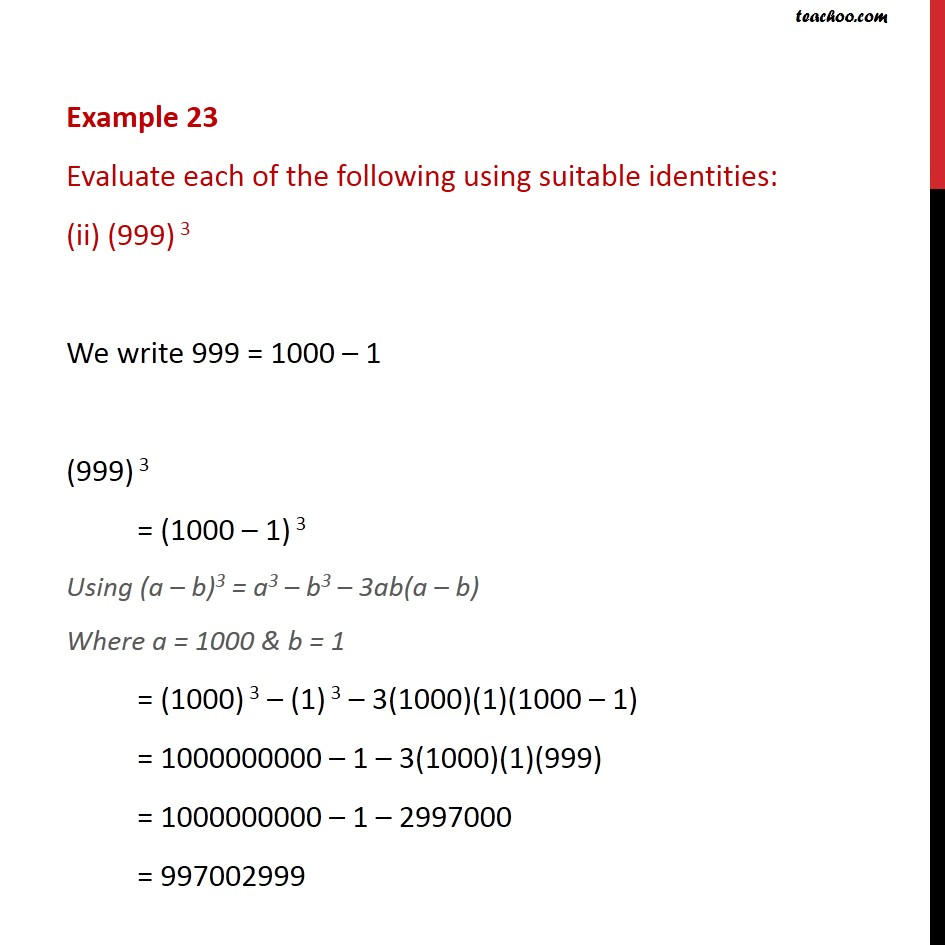Examples

Chapter 2 Class 9 Polynomials
Serial order wiseLearn in your speed, with individual attention - Teachoo Maths 1-on-1 Class

### Transcript

Example 23 Evaluate each of the following using suitable identities: (ii) (999) 3 We write 999 = 1000 – 1 (999) 3 = (1000 – 1) 3 Using (a – b)3 = a3 – b3 – 3ab(a – b) Where a = 1000 & b = 1 = (1000) 3 – (1) 3 – 3(1000)(1)(1000 – 1) = 1000000000 – 1 – 3(1000)(1)(999) = 1000000000 – 1 – 2997000 = 997002999